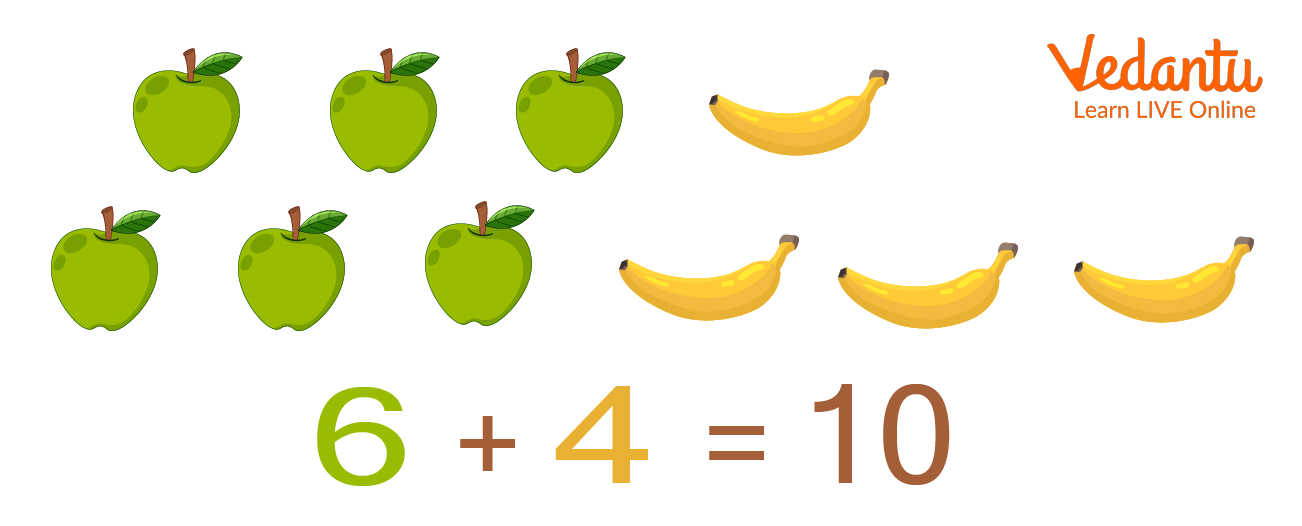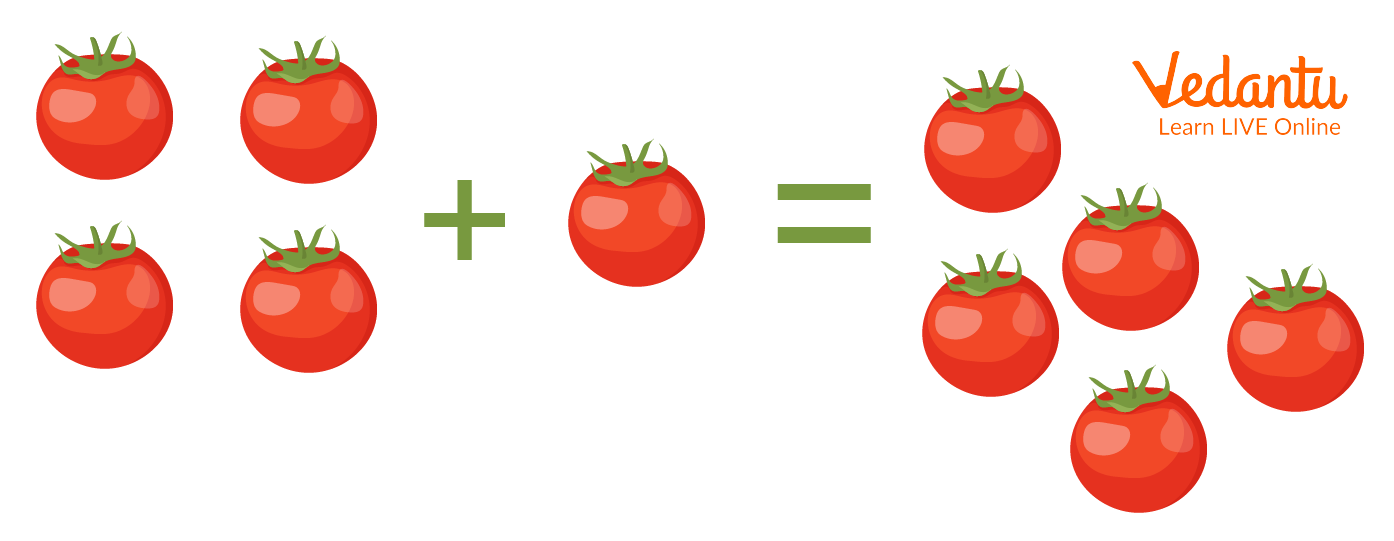Courses
Courses for Kids
Free study material
Free LIVE classes
More

# Addition Word Problems for Class 2 with Examples## An Introduction to Addition Word Problems for Class 2

Addition is the most basic mathematical operation that we can use for calculations. We need to practice using real-life situations and for that word problems are the best. Addition is based on certain properties, i.e. closure, commutative, and associative. Closure property is when two or more whole numbers are added to get a whole number. Commutative property is when two or more whole numbers are added but the result is not dependent on the order of addends. Associative property is when we add three or more numbers and the result obtained is the same and does not depend on the grouping of the addends.Addition of Two Types of Fruits

## Word Problems for Class 2 with Solution

In the below examples, we will see some word problems for Class 2 with solutions.

Example 1. Let us take two numbers 30 and 68. What is the sum of those two numbers?

Ans: One number = 30

Other number = 68

Therefore, sum of those two numbers = 30 + 68 = 98

Example 2. If there are 45 boys and 34 girls. What is the total number of students present in the classroom?

Ans: Number of boys in the classroom = 45

Number of girls in the classroom = 34

Therefore, total number of students in the classroom = 45 + 34 = 79

Example 3. Prithvi scored a brave 88 runs in the first match of the Ranji Trophy and scored 62 runs in the second match of the Ranji Trophy group stage. Find the total number of runs scored by him in the two matches.

Ans: Runs scored in first match= 88

Runs scored in the second match= 62

Total runs scored in those two matches = 88 + 62 = 150

2 + 8 = 10 so we carry 1

Now, 8 + 6 = 14 and we add that carried 1 here making it 15

So, the total is 150.

Example 4. There are 58 books on a bookshelf in the central library. 18 more books are kept on the same bookshelf later on. What is the total number of books on that shelf now?

Ans: Books on the bookshelf before = 58

Books on the bookshelf after the addition of new books= 18

Total number of books within the bookshelf = 58 + 18 = 76

8 + 8 = 16 so we carry 1

Now, 5 + 1 = 6 and we add that carried 1 here making it 7

So, the total is 76.

## Practice Problem Sums for Class 2

Q1. Shankar went to the local sweet shop and bought 31 Gulab Jamun. Her sister came home for Bhai Duj and brought 23 more Gulab jamun. How many Gulab jamun did Shankar have now altogether?

Ans: 54

Q2. Hema scored 43 marks out of 50 in a summative assessment. She scored 35 in marks on the final exam. How many marks does he get in all?

Ans: 78

Q3. Soham bought 31 pencils. His brother Ram has 46 pencils. How many pencils do they have altogether?

Ans: 77

##The Sum of 4 Tomatoes with 1

## Summary

In conclusion, we have learnt that addition is the most basic tool of calculation. This tool is the key to every other form of calculation. It is also a real-life skill that comes into use almost every day. Additional word problems are effective in making students understand the application of addition and clarify the concept by actual problems that they would face in life. These problems have everyday incidents regarding addition of objects such as clothes, food items, or toys. It can also relate to money where they calculate the total payable amount for the goods and items they purchase from a shop.

Last updated date: 19th Sep 2023
Total views: 113.1k
Views today: 2.13k

## FAQs on Addition Word Problems for Class 2 with Examples

1. Which properties of addition are necessary for adding more than one number?

Addition is used to combine different values together to obtain a final sum. The properties of addition which are used to add two or more numbers are closure, commutative and associative properties.

These properties make sure that addition is not dependent on the order or grouping of numbers and always give whole numbers as a result.

2. What are the uses of addition in our daily lives?

Addition is the combination of two different values that produced the total of them. We need to use addiction in various walks of our daily lives. A very common example of the use of addition is when we buy various grocery items from the grocery store, we have to know how much we need to pay the shopkeeper. We add the prices of the items we picked and the total sum is the amount of money that we pay.

3. What is the need for using word problems to teach kids?

The word problems present a real life scenario for the kids. They can put themselves in the position where they need to use addition to get the sum of objects, items or even money. This engages them very well than just adding numbers randomly and thus they learn better and more effectively. Word problems can present a very natural scenario that helps them to understand the different aspects and uses of addition.